Next: Degeneracy Up: Spin Previous: The Old Quantum Theory

#### The Hydrogen Atom, Then and Now

Let us look at a simple model of hydrogen atom: we neglect the spin of the proton and the electron, and relativistic effects. What remains is a point charge in an inverse-square force field-- the classical Kepler problem.

With these simplifications, the states of the hydrogen atom can be specified by giving three integer labels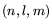, with: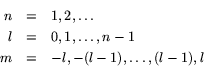The labelsare called quantum numbers, and made their debut in Sommerfeld's elliptical orbit model. They correspond to certain classical features of the orbit: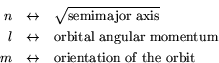Sommerfeld's quantum conditions stated that these three classical quantities were restricted to integer values (and in fact the collection of values given above).6 I should really say integer multiples of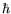'', but from now on I will adopt so-called natural units of measurement, which are chosen so that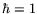.

I should make one refinement to this description of the old quantum'' viewpoint. The quantum conditions apply only to so-called stationary orbits. Bohr offered no details for what happened during a transition from one stationary orbit to another (a quantum jump''), nor could he explain why these orbits were stationary. Initially, physicists probably regarded these questions as topics for future research.

In the post-1925 reformulation, we have to solve a particular instance of Schrödinger's equation, subject to certain boundary conditions. The space of all solutions is a Hilbert space, with a basis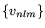. The vector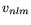is simultaneously an eigenvector of three operators: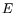, the energy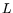, the magnitude of the orbital angular momentum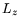, the-component of the orbital angular momentum

So we can say that if the hydrogen atom is in state, then it has a definite energy, and its angular momentum has a definite magnitude and-component. In fact, the eigenvalues forare: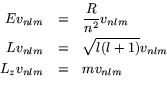where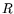is a physical constant known as Rydberg's constant.

The modern equivalent of the old notion of stationary orbit'' is eigenstate of the energy operator''. Such eigenstates do not change with time, and they possess a definite value for the energy. (These facts are closely related.) Schrödinger's equation, in fact, amounts to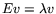, whereis the energy operator.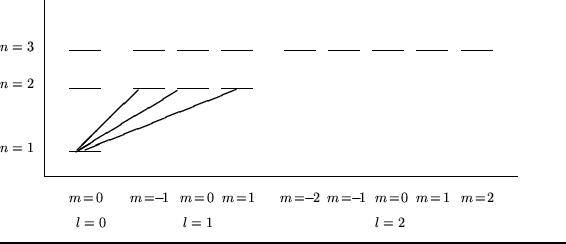Figure 1 gives a pictorial representation of the basisin a form known as a term scheme. The horizontal lines stand for basis vectors (or equivalently, stationary quantum states); height gives energy, and transitions are indicated by slanted lines. (Only three transitions are pictured, to avoid clutter.)

From this simple diagram, many treasures flow. The next few paragraphs give a taste.Next: Degeneracy Up: Spin Previous: The Old Quantum Theory